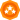Choose a Product Community
Resources

## Reading values from excel fileNew Contributor

## Reading values from excel file

My AUT exports excel files which contains dates and strings. I am trying to read the exported excel file but dates are read as encoded.
For instance, the excel has a column named Date and date format is 8/25/2016 11:13:52 AM but when I try to read this value with Excel object, I get

_x0038__x002F_25_x002F_2016_x0020_11:13:52_x0020_AM

How can I decode this to get the correct date format?

Code used to open and read excel file

var sheet = Excel.Open(filename).SheetByIndex(0);

sheet.Cell(7,2).Value;

4 REPLIES 4Champion Level 3New Contributor

This would not work for system which don't have Excel installed.Staff

Hi @newbiexiii -

Have you tried exporting the file to a .csv instead? It may have something to do with the formatting of the excel file.

EmmaContributor

Hi @newbiexiii.

Not sure what language you are coding, but the following JavaScript functions will show you how you can convert your date string.

The first is a generic function to convert hex codes to ascii characters. The second is specifically parsing your date string and then calling the hexToAscii function to get the ascii characters.  The third is just a test function.  You could combine them into one function if you wanted.

Have a look and let me know if this helps.

function hexToAscii(hexString)
{
// create an empty string
var ascii = "";
for (var i = 2; i < hexString.length; i += 2)
{
// get the substring, convert to int using base 16, then get the ascii character
ascii += String.fromCharCode(parseInt(hexString.substr(i, 2), 16));
}
return ascii;
}

function dateDecoder(excelValue)
{
// create a empty string to append and return
var retAscii = "";
// split the string on the underscore
var arr = excelValue.split('_');
// loop the array
for (var i = 0; i < arr.length; i++)
{
// if 5 characters and starts with 'x' then convert the hexcode to ascii otherwise just append the item to asciiString
if (arr[i].length == 5 && aqString.Find(arr[i], "x", 0, false) == 0)
retAscii += hexToAscii(aqString.Replace(arr[i], "x", "", false));
else
retAscii += arr[i];
}

return retAscii;
}

function decoderTest()
{

BuiltIn.ShowMessage(dateDecoder("_x0038__x002F_25_x002F_2016_x0020_11:13:52_x0020_AM"));
}

Chriscancel
Showing results for